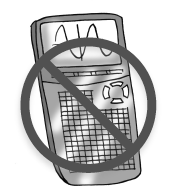### Home > CC4 > Chapter 1 > Lesson 1.2.1 > Problem1-41

1-41.

Perform the indicated operation to evaluate each expression. Homework Help ✎1. $- \frac { 1 } { 2 } + ( - \frac { 1 } { 5 } )$

Adding or subtracting fractions requires common denominators.
What number is the Least Common Multiple of $2$ and $5$?

1. $-\frac{2}{3}-2$

What is the denominator for the integer $2$?

1. $-1\frac{2}{3}\left(-2\right)$

Try changing the mixed number to a fraction greater than 1.

${-1\frac{2}{3}(-2)}$
${\frac{-5}{3}\cdot \frac{-2}{1}}$

1. $-2\ \div \frac{2}{3}$

Dividing by a fraction is the same as multiplying by the reciprocal of the fraction.

$−3$